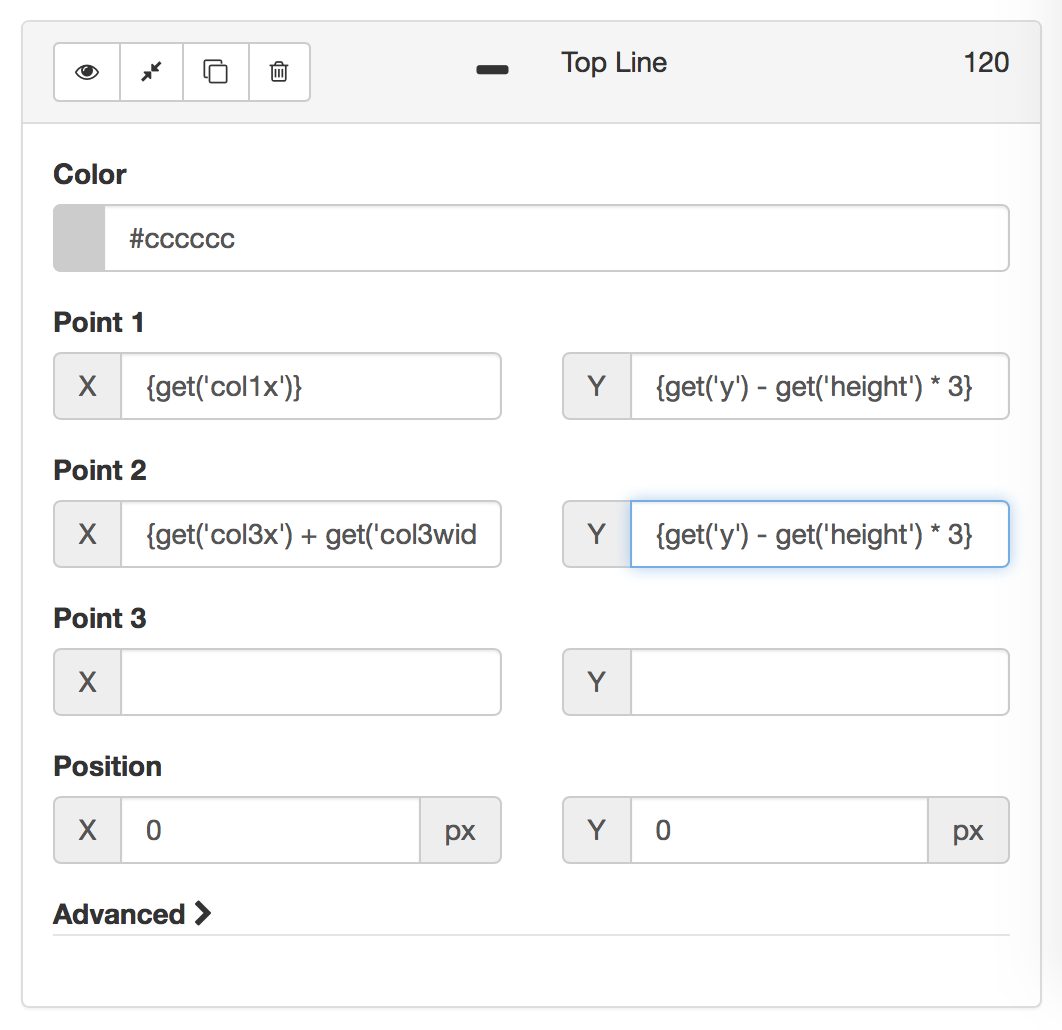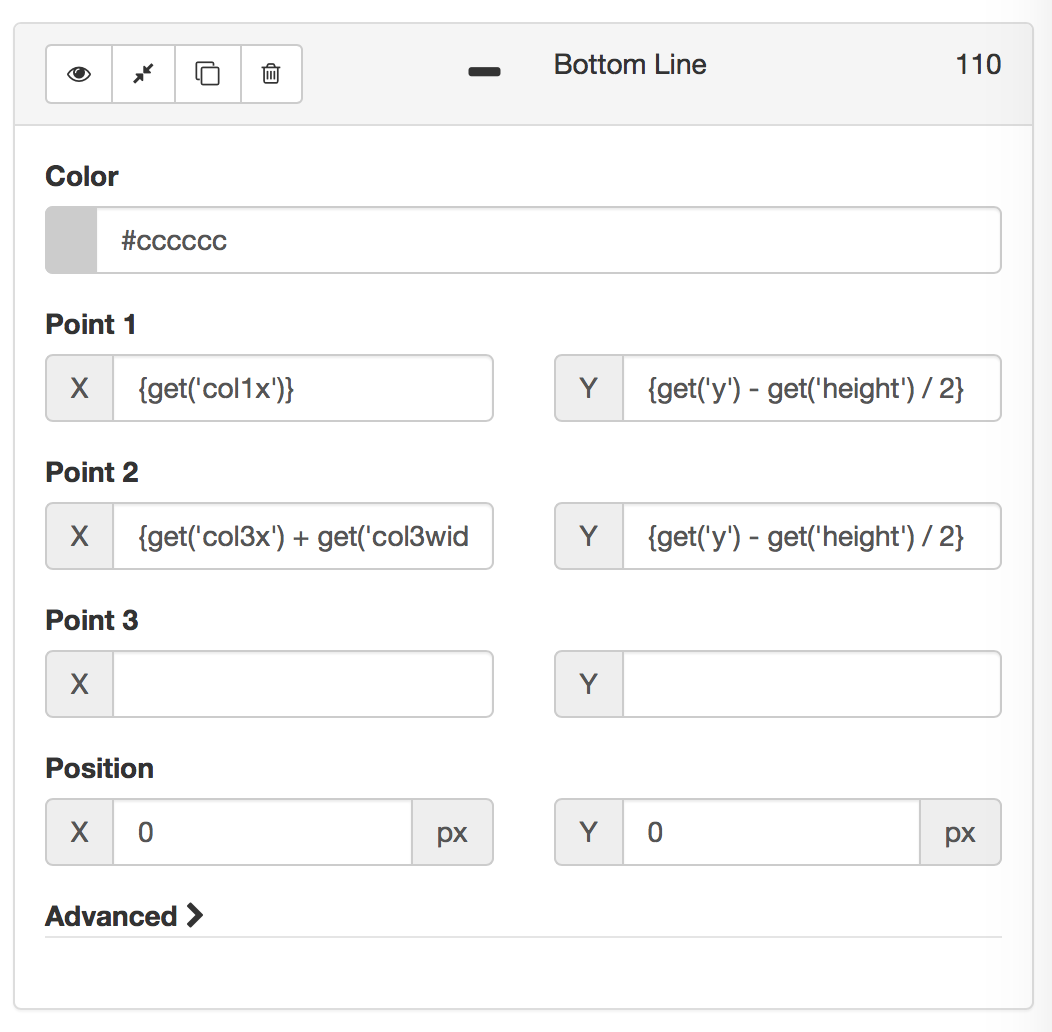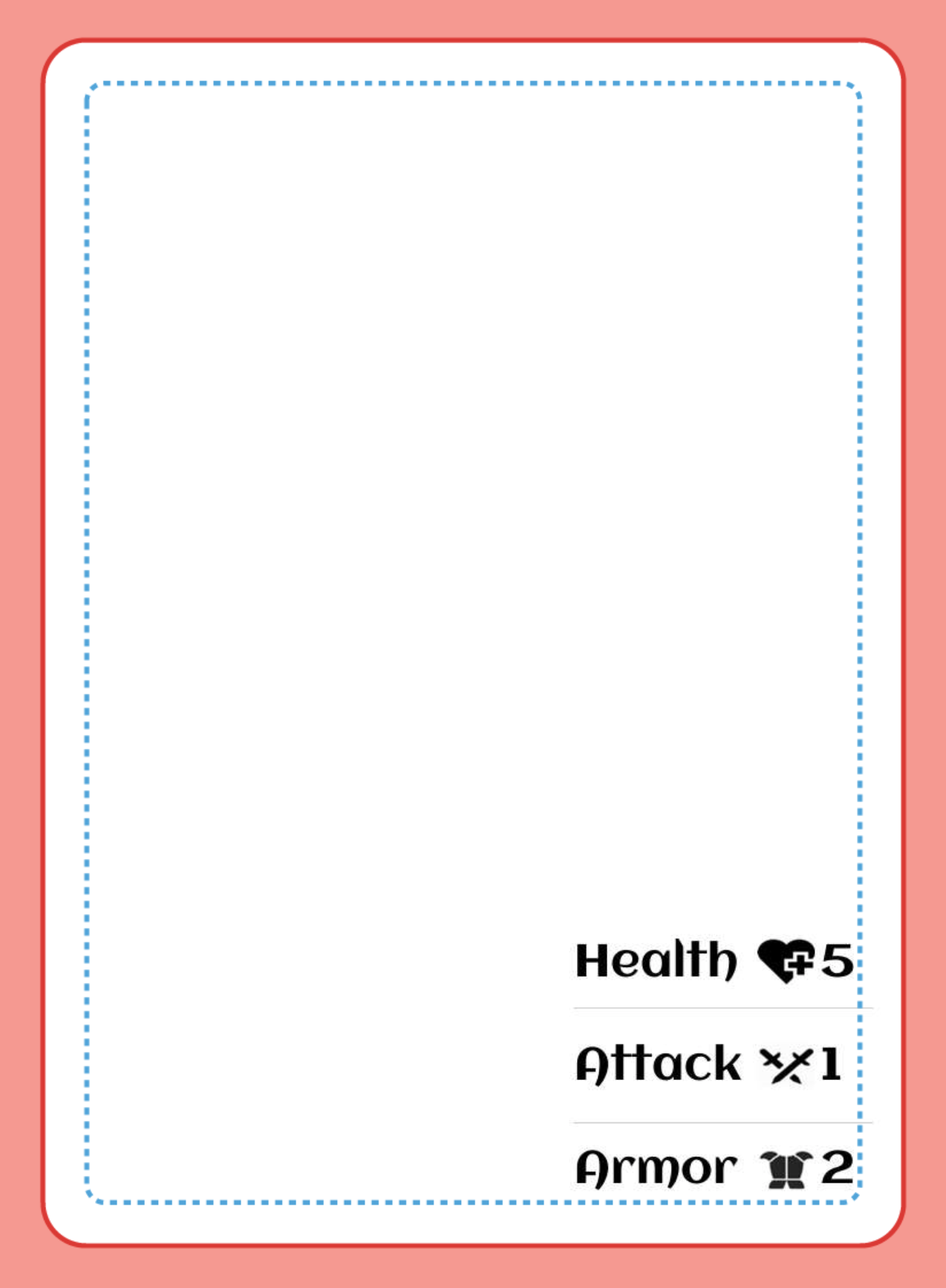# [CS1] Dynamic Tables / Charts

Sometimes you need to build a table or chart of text and/or icons. For the purposes of this example, let's draw a 3 x 3 table where the first column is text, the second column is an icon, and the third column is text again.

We could do this by simply specifying where to position everything. However, by using a Note step we can set up variables, such that if we need to change the width of the columns, or the height of the rows then we'd only need to make small changes to the initial variables, and our changes will be reflected across our entire component. So for this example, we'll be using the Note step.

Start by adding a note and setting variables for your current y position, and the x position of each column, as well as the width of each column:

` { set('y', 100) set('height',50) set('margin', 10) set('col1x', 100) set('col1width',150) set('col2x', get('margin') + get('col1x') + get('col1width')) set('col2width',50) set('col3x', get('margin') + get('col2x') + get('col2width')) set('col3width',50) } `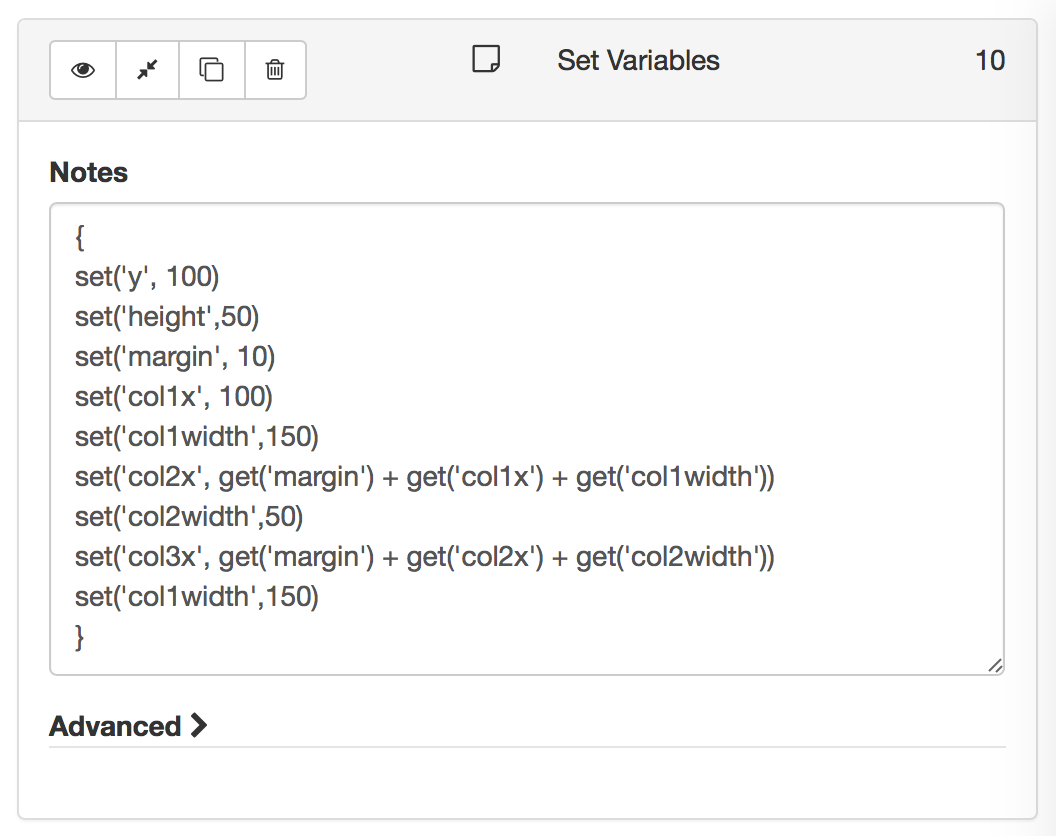Now you can draw your first cell. Use Add Text, and set the position to `{get('col1x')}`, `{get('y')}`. Set the size to `{get('col1width')}`, `{get('height')}`.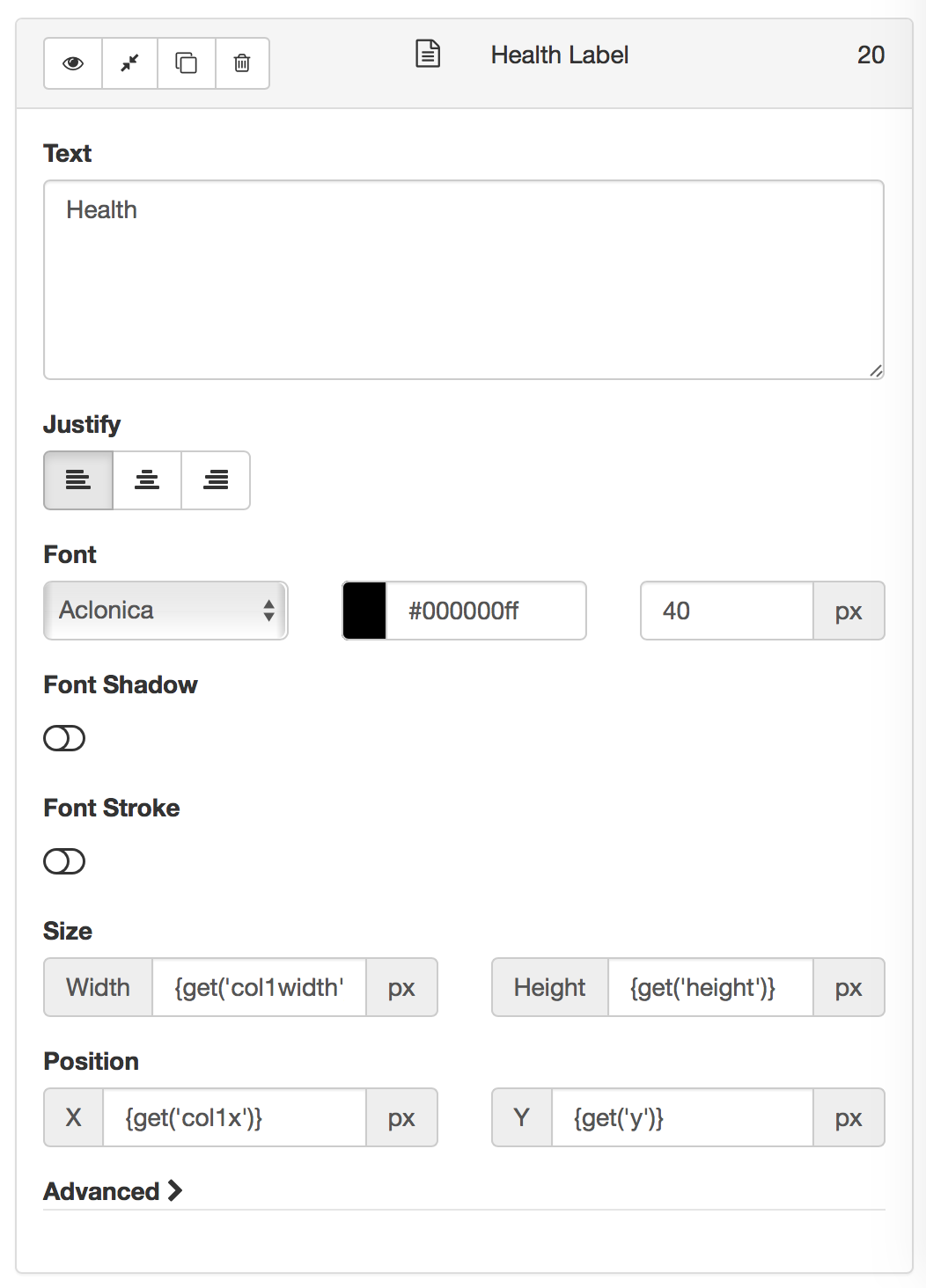The second cell will use Add Image, and set the position to `{get('margin') + get('col2x')}`, `{get('y')}`. Set the resize to `{get('col2width')}``{get('height')}`.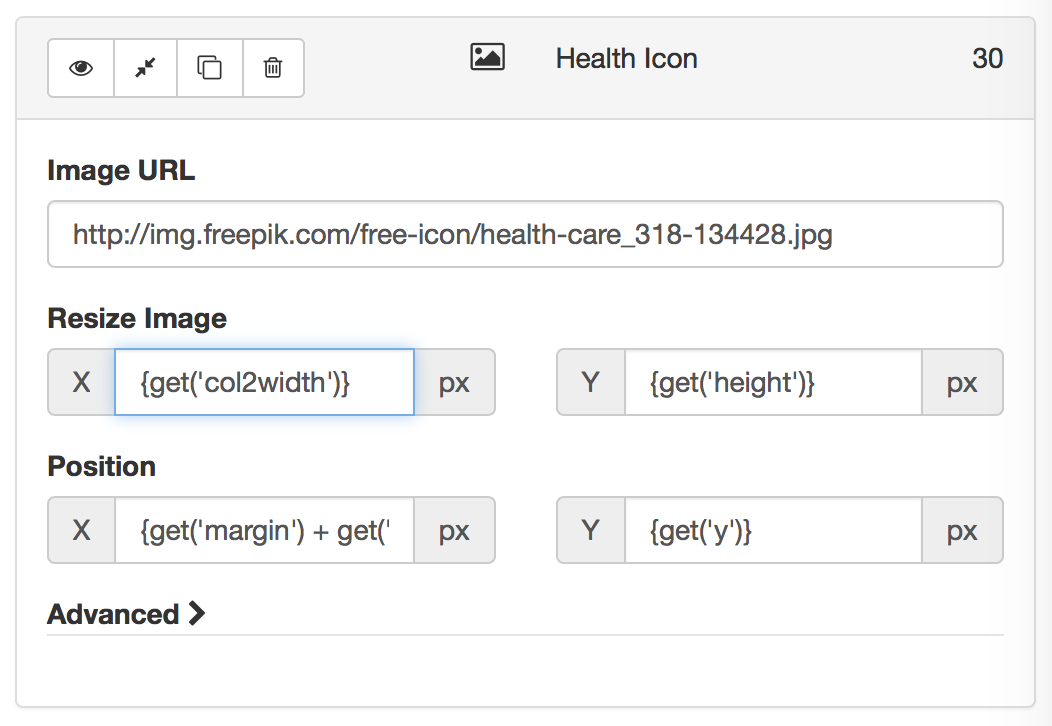And finally we can draw the last cell using Add Text, and set the position to `{get('margin') + get('col3x')}`, `{get('y')}`. Set the size to `{get('col3width')}`, `{get('height')}`.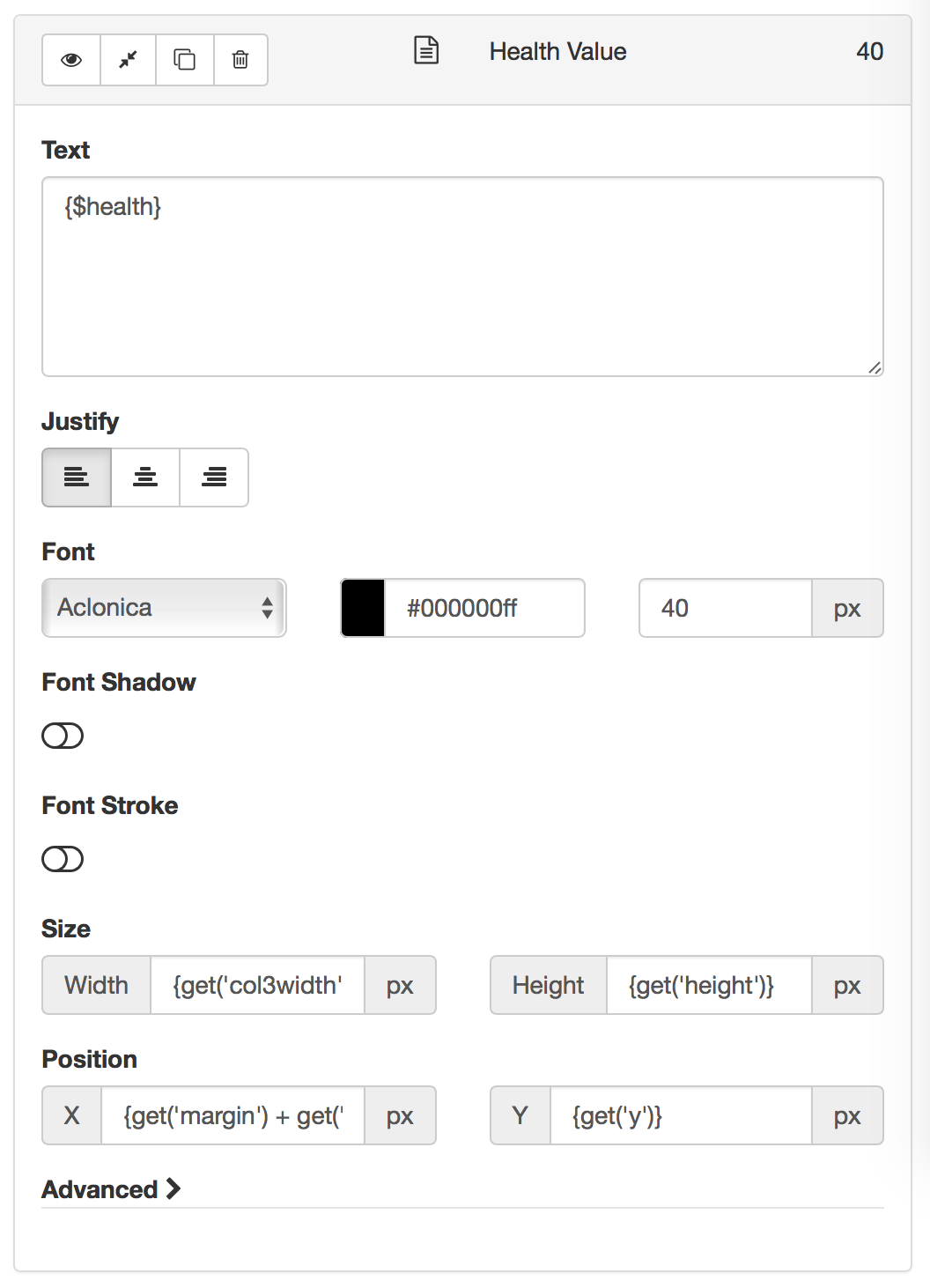Here's what we've got so far:We're ready to make our next row. We could use a note to update our y position, but there's a fancy way to do it inline using `{setget()}`, so we'll do that instead.

Draw your first cell in the new row using Add Text, and set the position to `{get('col1x')}`, `{setget('y',get('y') + get('margin') + get('height'))}`. Set the size to `{get('col1width')}`, `{get('height')}`. By using setget() here we have incremented the y value by margin + height and we have also returned the new value into the y field.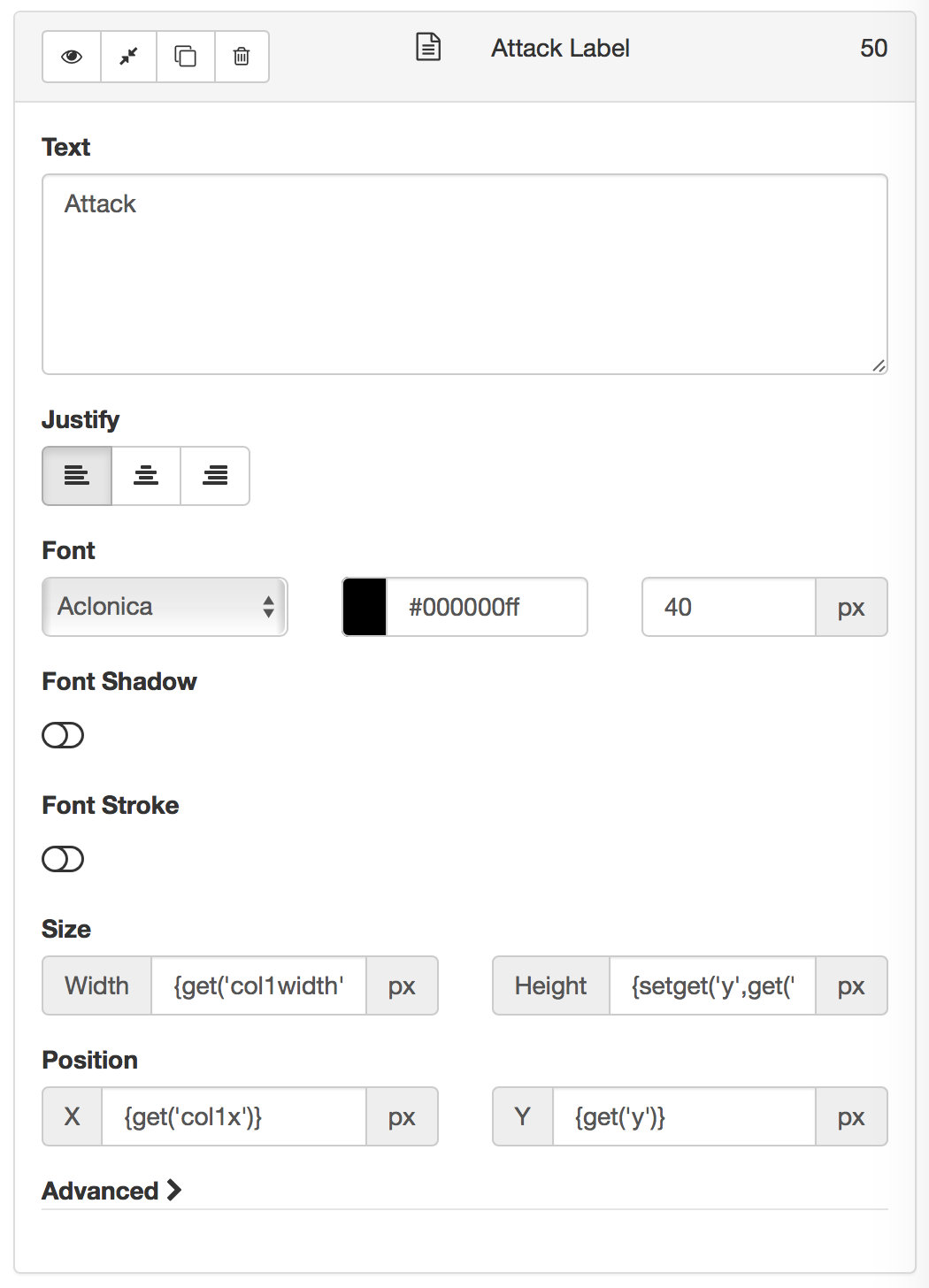The second sell will use Add Image again, and set the position to `{get('margin') + get('col2x')}`, `{get('y')}`. Set the resize to `{get('col2width')}`, `{get('height')}`.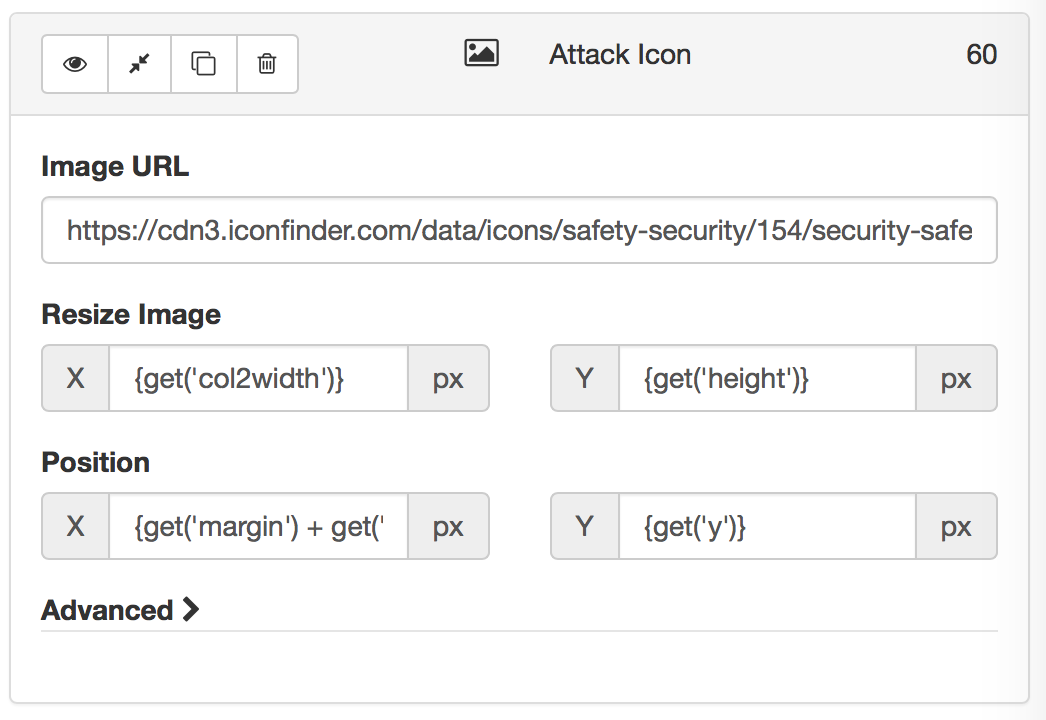And finally we can draw the last cell using Add Text, and set the position to `{get('margin') + get('col3x')}`, `{get('y')}`. Set the size to `{get('col3width')}`, `{get('height')}`.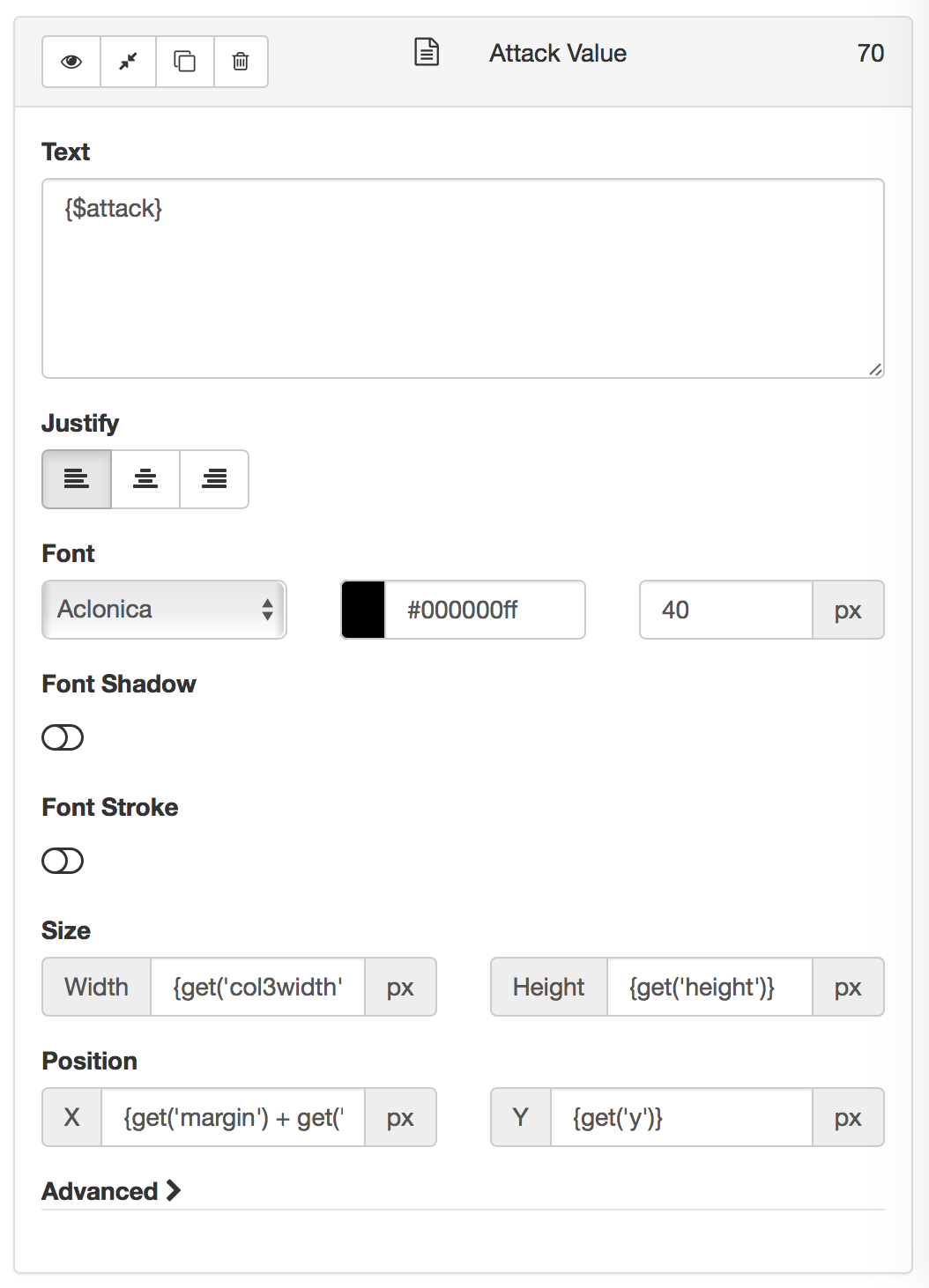Here's what our table looks like to this point: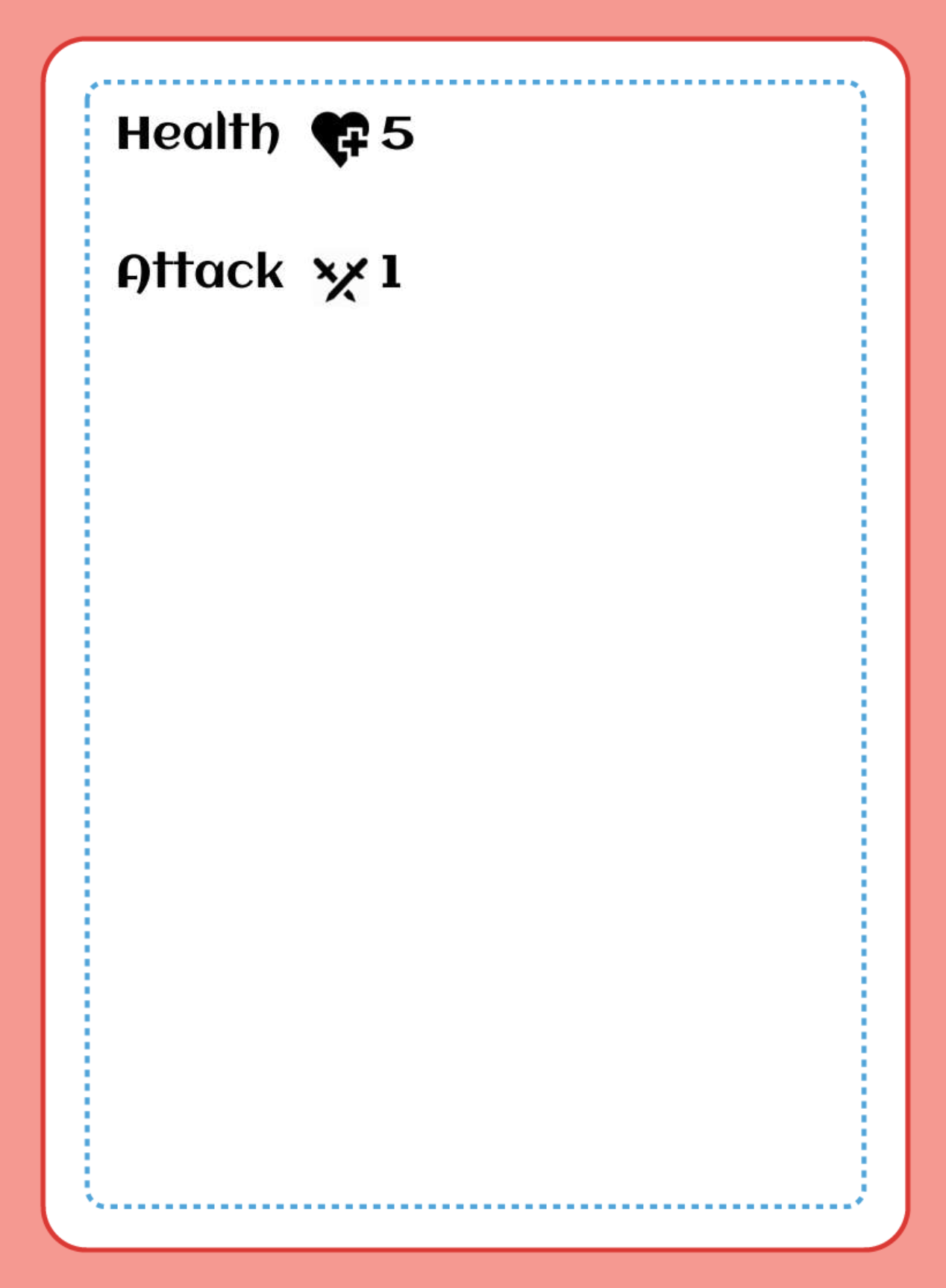A keen observer will note that that column 1 of this row was nearly identical to column 1 in the first row, and columns 2 and 3 are absolutely identical. Therefore using the duplicate tool is a great idea to create these, and we'll do exactly that for the next 3 columns.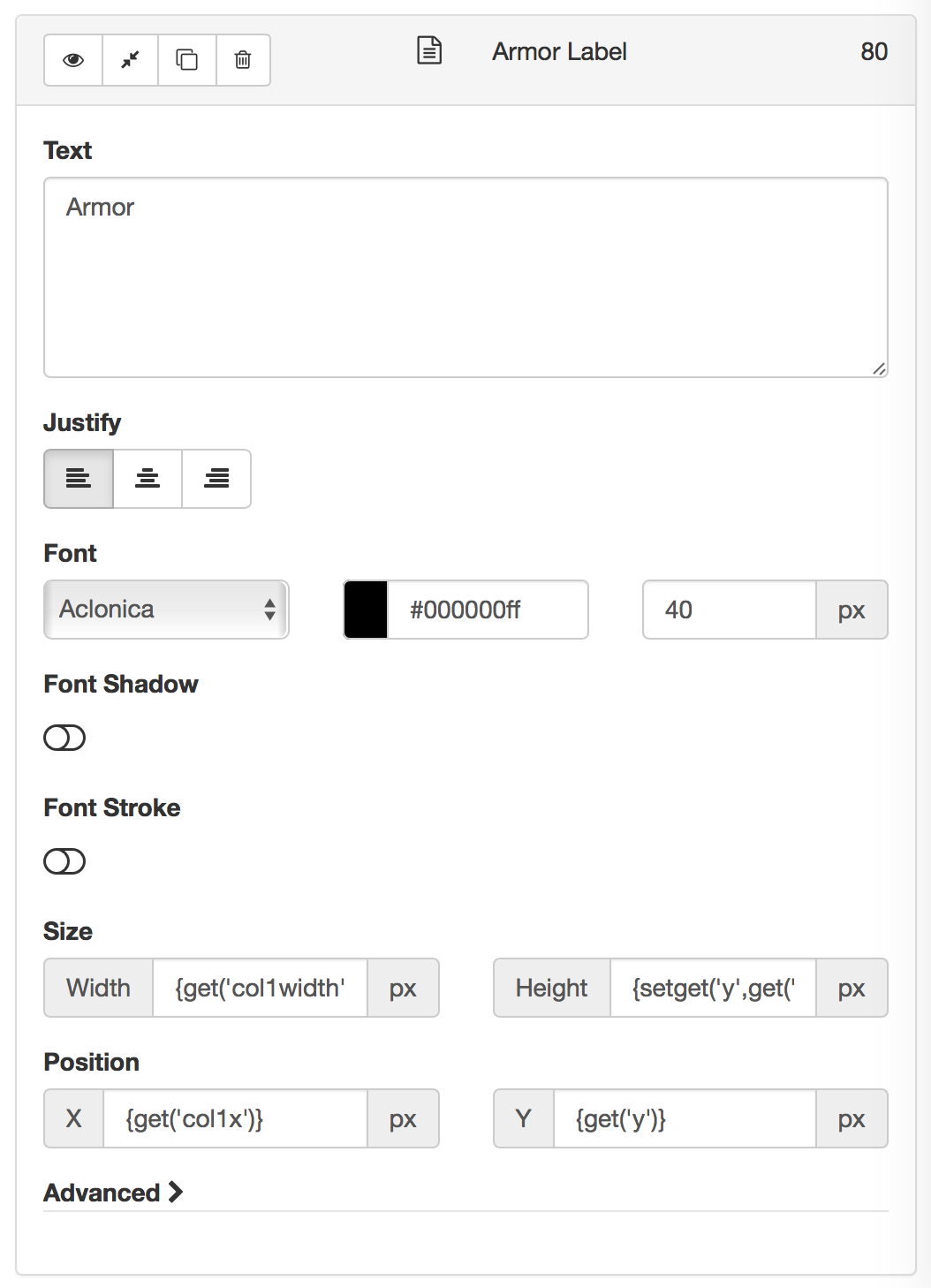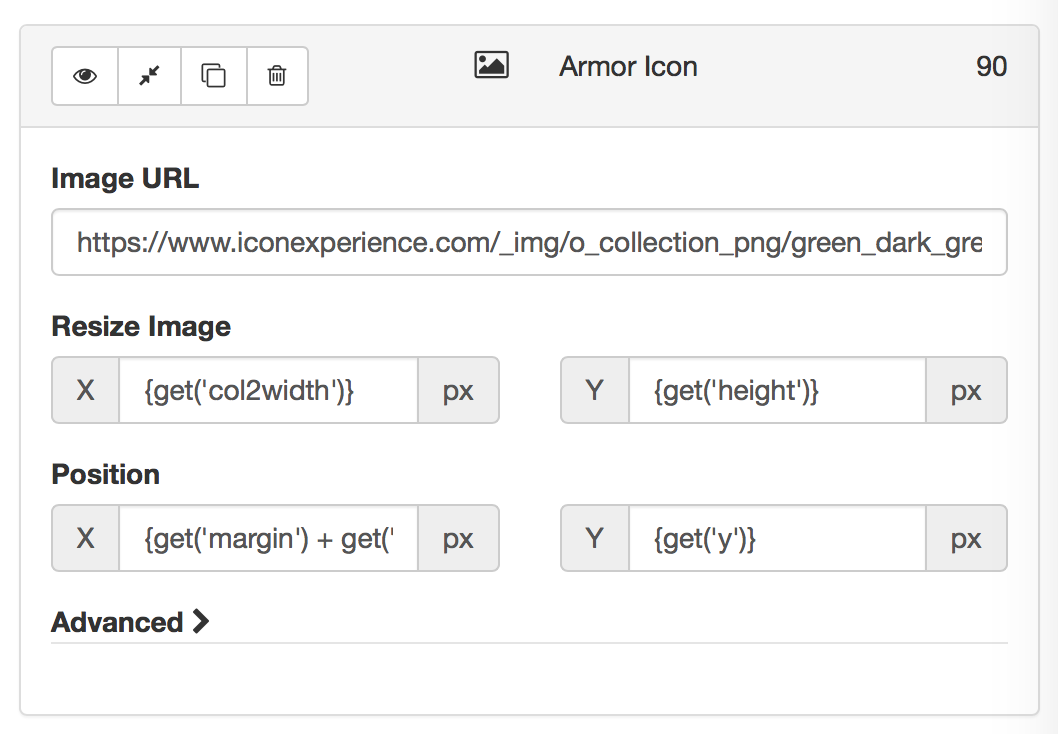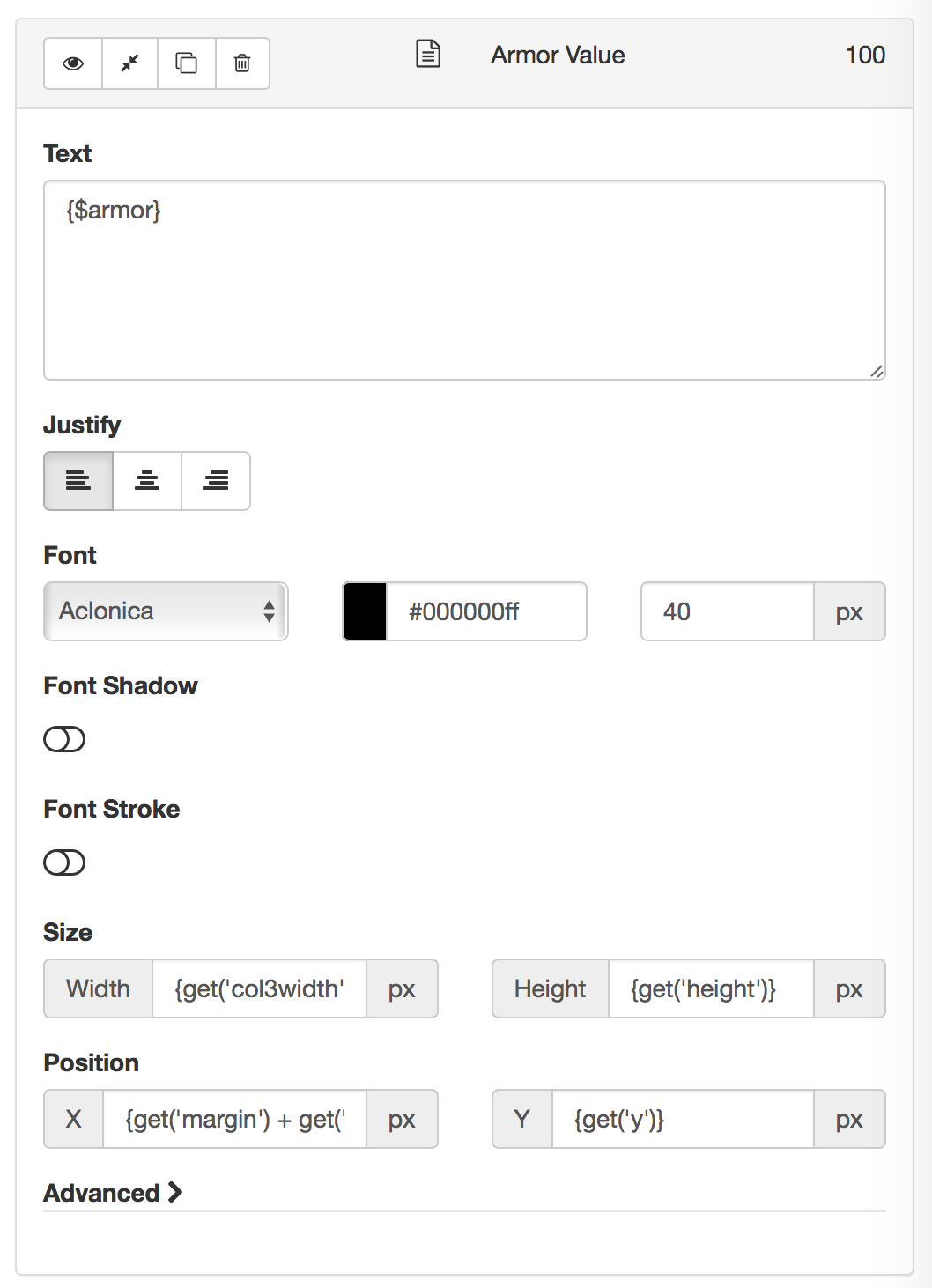So here is our table: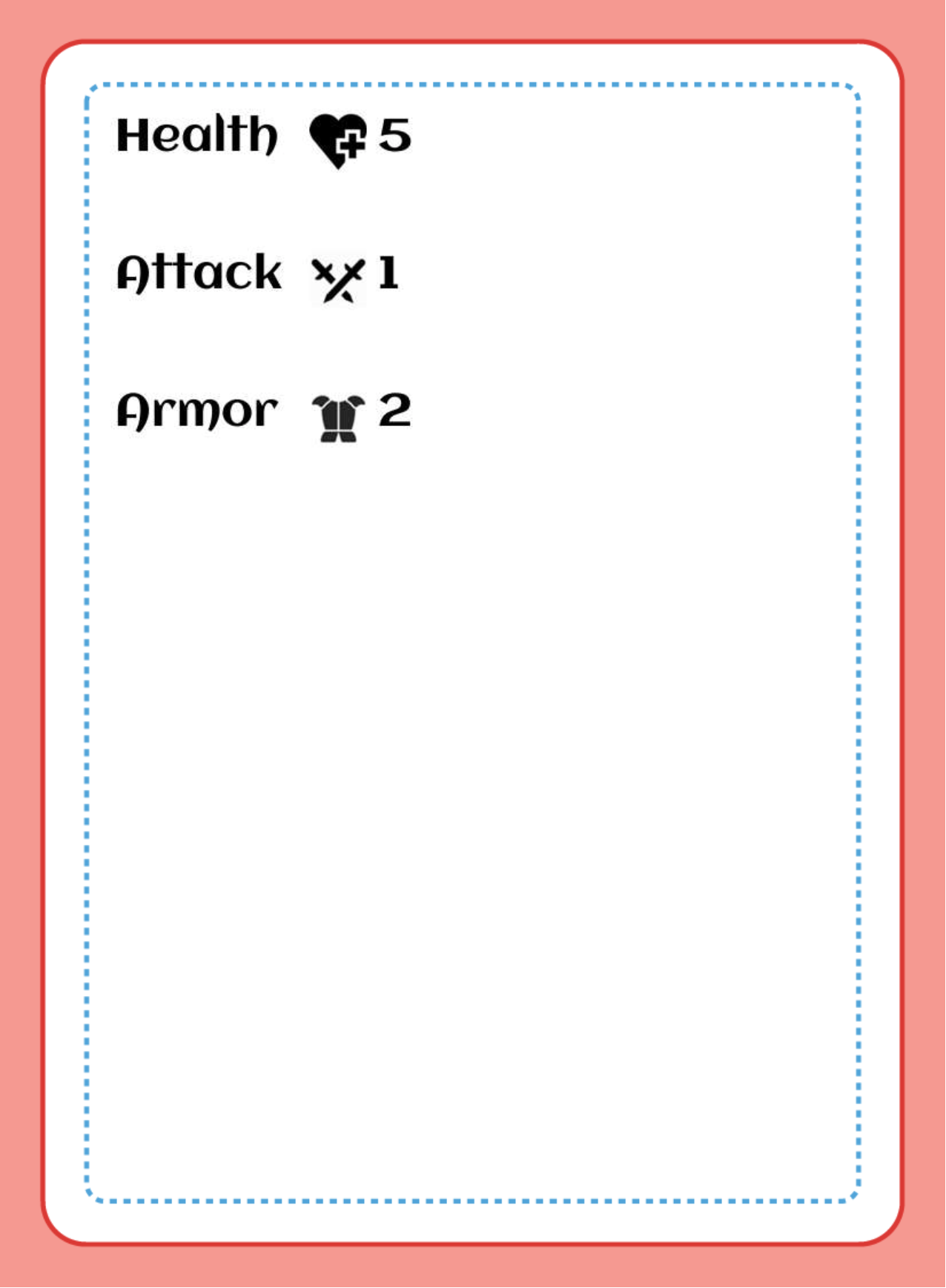We might decide that the rows are slightly too far apart, and that we want our table in the lower right corner of the card. If we had hard coded everything then these changes would be a pain to make. However, since we have put everything into variables in the note field at the top of the design, we can just modify a few variables.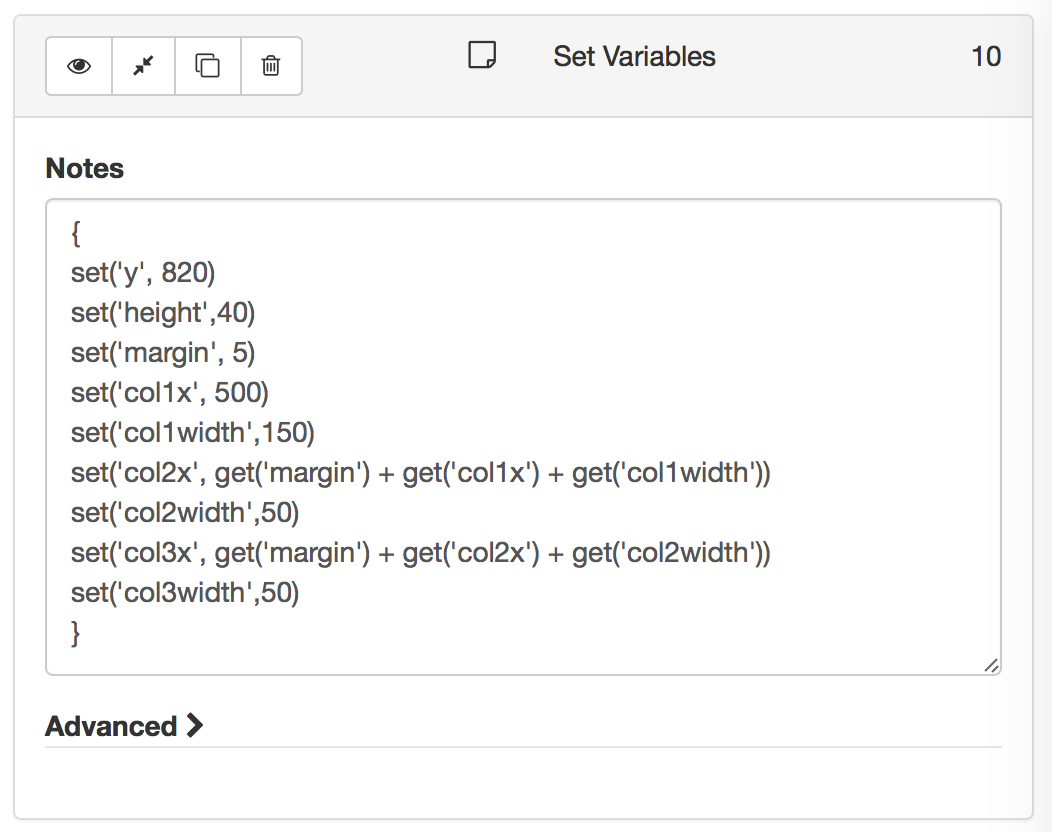And now our table looks like this: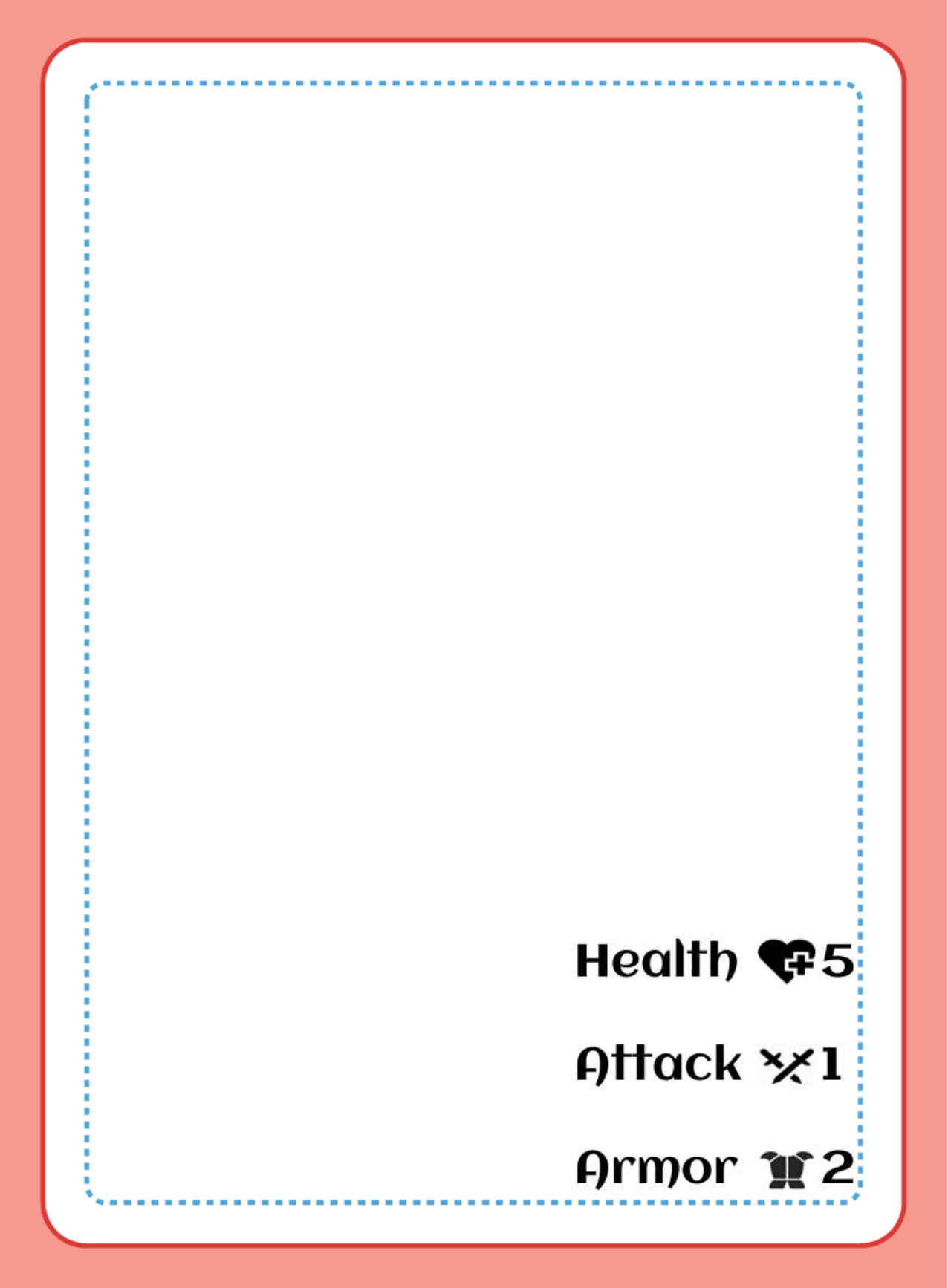And if we want, we could now even get a bit fancy and add in some lines to separate things a bit.